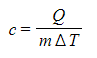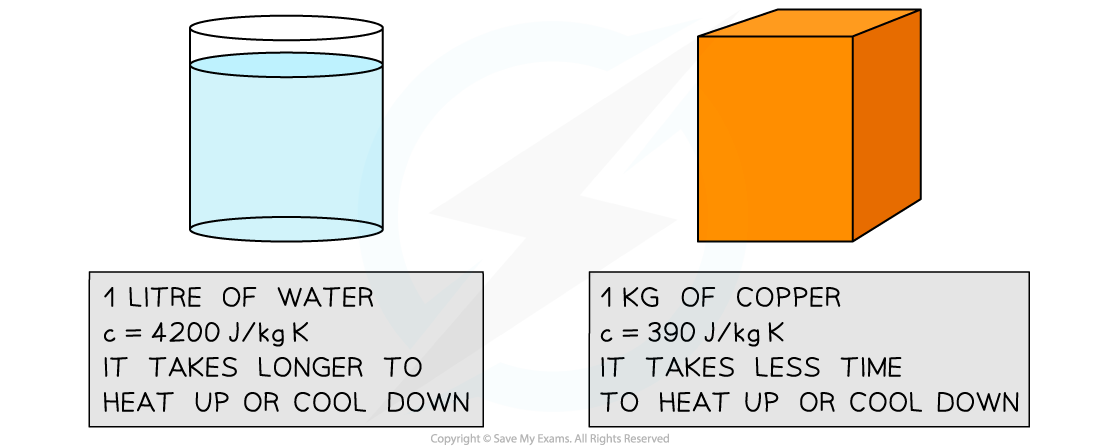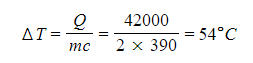# IB DP Physics: SL复习笔记3.1.4 Specific Heat Capacity

### Specific Heat Capacity

• The amount of thermal energy needed to change the temperature of an object depends on:
• The change in temperature required ΔT - i.e. the larger the change in temperature the more energy is needed
• The mass of the object m - i.e. the greater the mass the more energy is needed
• The specific heat capacity c of the given substance - i.e. the higher the specific heat capacity the more energy is needed• The equation for the thermal energy transferred, Q, is then given by:

Q = mcΔT

• Where:
• m = mass of the substance in kilograms (kg)
• ΔT = change in temperature in kelvin (K) or degrees Celsius (°C)
• c = specific heat capacity of the substance (J kg–1 K–1)
• The specific heat capacity of a substance is defined as:

The amount of energy required to change the temperature of 1 kg of a substance by 1 K (or 1°C)

• This definition can be explained when the above equation is rearranged for c:
•This means that, the higher the specific heat capacity of a substance the longer it takes for the substance to warm up or cool down
• Note that the specific heat capacity is measured in J kg–1 K–1#### Worked Example

A 2 kg piece of copper is kept inside a freezer at a temperature of –10°C. The copper is taken out of the freezer and placed into 5 litres of water at 20°C. A thermometer is placed into the water. After some time, the thermometer indicates that the water has cooled to 18°C.Determine the temperature of the copper at this time. Give your answer in degrees Celsius (°C).

• The specific heat capacity of water is 4200 J kg–1 K–1
• The specific heat capacity of copper is 390 J kg–1 K–1

Step 1: Write down the known quantities

• Mass of copper = 2 kg
• Mass of water = 5 L = 5 kg
• Initial temperature of copper = –10°C
• Initial temperature of water = 20°C
• Final temperature of water = 18°C
• Change in temperature of water = 18°C – 20°C = –2°C
• Specific heat capacity of water = 4200 J kg–1 K–1
• Specific heat capacity of copper = 390 J kg–1 K–1

Step 2: Write down the equation for thermal energy

Q = mcΔT

Step 3: Determine the energy transferred from the water to the copper

• The water is at a higher temperature than copper, hence thermal energy will flow from the water to the copper
• To quantify this energy, substitute numbers into the above equation
• In this case, the mass m is that of the water
• The specific heat capacity is that of water
• Since this is the energy lost by the water, it will be negative

Q = 5 kg × 4200 J kg–1 K–1 × (–2°C) = – 42000 J

Step 3: Determine the change in temperature ΔT of the copper

• The energy lost by the water is the same as the energy gained by the copper
• Since this is the energy gained by the copper, it is positive
• The equation for thermal energy can be rearranged to calculate the change in temperature ΔT of the copper
• In this case, the mass m is that of the copper
• The specific heat capacity is that of copperStep 4: Determine the final temperature of the copper

• Since the copper gains thermal energy, its final temperature will be higher than its initial temperature

final temperature of copper = ΔT + initial temperature of copper = 54°C – 10°C

final temperature of copper = 44°C

#### Exam Tip

You should notice that changes in temperature ΔT can usually be written in degrees Celsius (although this is not the SI base unit for temperature) and do not need to be converted into kelvin (K). This is because differences in absolute temperatures always correspond to differences in Celsius temperature.If the question asks to determine the initial or final temperature of a substance, make sure you always check the unit of measure (°C or K) in which you are required to give your final answer.